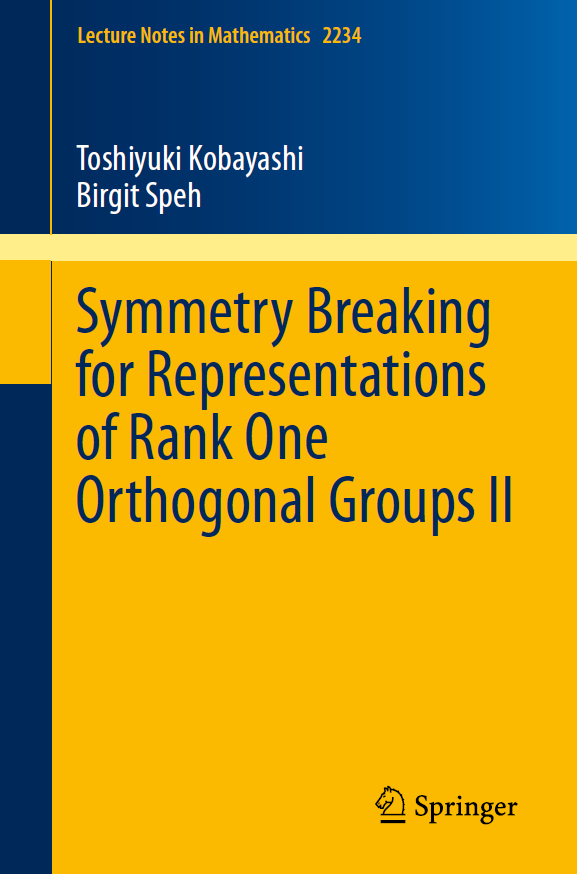## T. Kobayashi and B. Speh. Symmetry Breaking for Representations of Rank One Orthogonal Groups II, volume 2234 of Lecture Notes in Mathematics. Springer, 2018. xv+342 pages, ISBN: 978-981-13-2900-5, eBook: 978-981-13-2901-2. arXiv: 1801.00158. DOI: 10.1007/978-981-13-2901-2..For a pair (G,G')=(O(n+1,1), O(n,1)) of reductive groups, we investigate intertwining operators (symmetry breaking operators) between principal series representations Iδ(V,λ) of G, and Jε(W,ν) of the subgroup G'. The representations are parametrized by finite-dimensional representations V, W of O(n) respectively of O(n-1), characters δ, ε of O(1), and λ, νinC. Denote by [V:W] the multiplicity of W occurring in the restriction V|O(n-1), which is either 0 or 1. If [V:W] =/=0 then we construct a holomorphic family of symmetry breaking operators and prove that C HomG'(Iδ(V, λ)|G', Jε(W, ν)) is nonzero for all the parameters λ, ν and δ, ε, whereas if [V:W] = 0 there may exist sporadic differential symmetry breaking operators.

We propose a classification scheme to find all matrix-valued symmetry breaking operators explicitly, and carry out this program completely in the case (V,W)=(i(Cn), j(Cn-1)). In conformal geometry, our results yield the complete classification of conformal covariant operators from differential forms on a Riemannian manifold X to those on a submanifold Y in the model space (X, Y) = (Sn, Sn-1).

We use this information to determine the space of symmetry breaking operators for any pair of irreducible representations of G and the subgroup G' with trivial infinitesimal character. Furthermore we prove the multiplicity conjecture by B. Gross and D. Prasad for tempered principal series representations of (SO(n+1,1), SO(n,1)) and also for 3 tempered representations Π, π, ϖ of SO(2m+2,1), SO(2m+1,1) and SO(2m,1) with trivial infinitesimal character. In connection to automorphic form theory, we apply our main results to find periods of irreducible representations of the Lorentz group having nonzero (g, K)-cohomologies.

This book is an extension of the recent work in the two research monographs: Kobayashi-Speh [Memoirs Amer. Math. Soc., 2015] for spherical principal series representations and Kobayashi-Kubo-Pevzner [Lecture Notes in Math., 2016] for conformally covariant differential symmetry breaking operators.